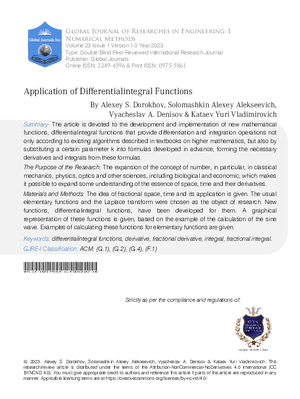### Application of Differentialintegral Functions

Authors

• Alexey S. Dorokhov

• Solomashkin Alexey Alekseevich

• Vyacheslav A. Denisov

Keywords:

differentialintegral functions, derivative, fractional derivative, integral, fractional integral

Abstract

The article is devoted to the development and implementation of new mathematical functions differentialintegral functions that provide differentiation and integration operations not only according to existing algorithms described in textbooks on higher mathematics but also by substituting a certain parameter k into formulas developed in advance forming the necessary derivatives and integrals from these formulas The Purpose of the Research The expansion of the concept of number in particular in classical mechanics physics optics and other sciences including biological and economic which makes it possible to expand some understanding of the essence of space time and their derivatives Materials and Methods The idea of fractional space time and its application is given The usual elementary functions and the Laplace transform were chosen as the object of research New functions differentialintegral functions have been developed for them A graphical representation of these functions is given based on the example of the calculation of the sine wave Examples of calculating these functions for elementary functions are given Of particular interest is the differentialintegral function in which the parameter k is a complex number s s a i b although in general the parameter k can be any function of a real or complex argument as well as the differentialintegral function itself Research Results As a result of the research it is shown how the Laplace transform and Borel s theorem are used to calculate differentialintegral functions It is shown how to use these functions to carry out differentiation and integration It is presented how fractional derivatives and fractional integrals should be obtained Dependencies for their calculation are obtained Examples of their application for such functions as cos x exp x and loudness curves in music Fletcher-Manson or Robinson-Dadson curves are shown Conclusions Studies show the possibility of a wide application of differentialintegration functions in modern scientific research These functions can be used both in office and in specialized programs where calculations of fractional derivatives and fractional integrals are needed

How to Cite

Alexey S. Dorokhov, Solomashkin Alexey Alekseevich, Vyacheslav A. Denisov, & Kataev Yuri Vladimirovich. (2023). Application of Differentialintegral Functions. Global Journals of Research in Engineering, 23(I1), 7–32. Retrieved from https://engineeringresearch.org/index.php/GJRE/article/view/101578Published

2023-04-27

Issue

Section

Articles